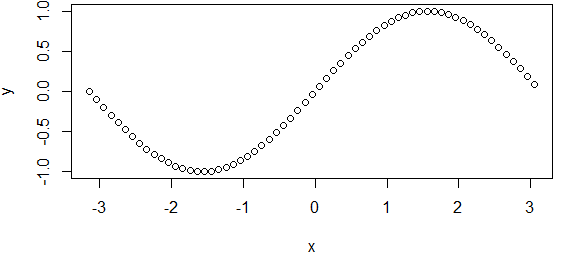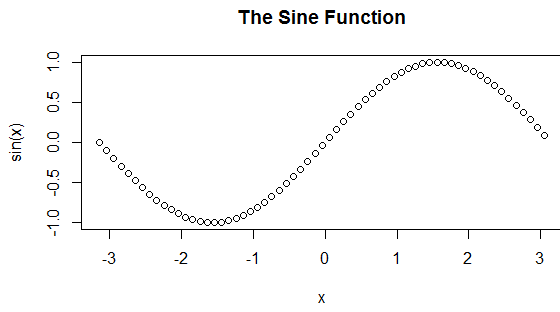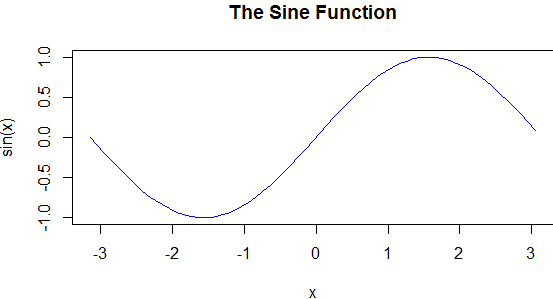Showing posts with label r plot example program. Show all posts
Showing posts with label r plot example program. Show all posts

## r plot program example and how to use it.

R Plot Function is used to plot a graph in r programming in this r function we can vector and then we get a graph which is about  magnitude vs index.

r plot function has so many method which is called according to the passed value to this r plot function.

For example we pass two vector plot(c(1,2),c(3,5)) and then this function plot a scatter graph for these two point.

here we plot graph for sine function form range -pi to pi as you can see in this picture.

```<- pi="" plot="" pre="" seq="" sin="" x="">```x <- seq(-pi,pi,0.1)
plot(x, sin(x))``````R Plot Function

# Adding Titles and Labeling Axes to the r plot function

Here you can add title and label to the with parameter main and xlab and ylab two parameter for labeling x-axis and y-axis in plot graph.

``````plot(x, sin(x),
main="The Sine Function",
ylab="sin(x)")``````Labeling Axes to the r plot function

# Changing Color and Plot Type for r function : -

Well you can change the color for plot type with the help of the argument type in r languages.

Check out the example for r plot with color:-

``````plot(x, sin(x),
main="The Sine Function",
ylab="sin(x)",
type="l",
col="blue")``````

here we can define the color with colchanging Color and Plot Type